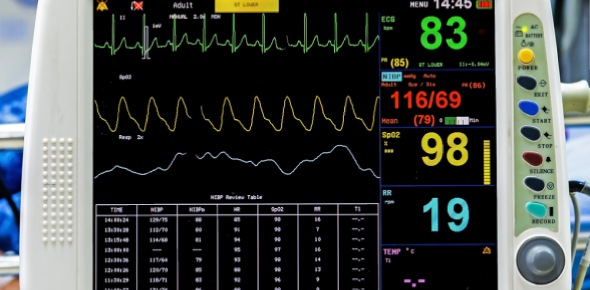# EKG Set Up And Leads

36 Questions | Attempts: 526
ShareSettings• 1.
What is an ECG?
• A.

A graphic representaion

• B.

Measures electrical impulses originating form the myocardium

• C.

A specualized recording device that uses electrodes

• D.

Rate, rhythm, conduction

• E.

All of the above

• 2.
Why do doctors order ECG's?
• A.

Cost efficient

• B.

Non-invasive procedure

• C.

Quick and accurate results

• D.

Essential for diagnoses and treatment

• E.

to give the heart a jump on electrical impulses

• 3.
What wave shows Right and Left Atrial depolerization
• A.

P wave

• B.

QRS wave

• C.

T wave

• D.

U wave

• E.

QT interval

• 4.
What wave shows Right and Left Ventricular depolerization?
• A.

P wave

• B.

QRS wave

• C.

T wave

• D.

U Wave

• E.

ST segment

• 5.
In what wave does Atrial repolerization happen?
• A.

P wave

• B.

QRS wave

• C.

T wave

• D.

Uwave

• 6.
What wave shows ventricular repolerization?
• A.

P wave

• B.

QRS wave

• C.

T wave

• D.

U wave

• 7.
What wave is not always present?
• A.

P wave

• B.

QRS wave

• C.

T wave

• D.

U wave

• 8.
What is the PR interval?
• A.

Distance between the end of the P wave to the beggining of the QRS wave

• B.

Distance between the end of the P wave and the end of the QRS wave

• C.

Distance between the begining of the P wave and the end of the QRS wave

• D.

Distance between the begining of the P wave and the beginning of the QRS wave

• 9.
QT interval is the ____ ?
• A.

Distance between the begining of the QRS wave and the end of the T wave

• B.

Distance between the end of the QRS wave and the end of the T wave

• C.

Distance between the end of the QRS wave and the begining of the T wave

• D.

Distance between the begining of the QRS and the begining of the T wave

• 10.
ST segment is the ____?
• A.

Distance between the begining of the QRS to the begining of the T wave

• B.

Distance between the begining of the QRS to the end of the T wave

• C.

Distance between the end of the QRS to the end of the T wave

• D.

Distance between the end of the QRS to the begining of the T wave

• 11.
When the machine is checking frequency response of impulses. This shows as a squared off segment on the strip
• A.

Calibration pulse

• B.

Standardization pulse

• C.

Setting pulse

• D.

F.S. pulse

• E.

Frequnecy pulse test

• 12.
Each vertical square on the graph paper represents____ and is measured in _____ mV
• A.

Voltage, 0.04

• B.

Voltage , 0.1

• C.

Time, 0.04

• D.

Time, 0.1

• 13.
Each horizontal small block represents ______, and is in _____mm/sec
• A.

Voltage , 0.04

• B.

Voltage, 0.1

• C.

Time , 0.04

• D.

Time, 0.1

• 14.
Cardiac activity is recorded on ECG paper and is shown in ?
• A.

Peaks and valleys

• B.

Hight and width

• C.

Waves and deflection

• 15.
Wave heights are also known as?
• A.

Peaks

• B.

Amplitudes

• C.

Interval widths

• 16.
Paper generally runs through the machine at ____ mm/sec
• A.

20mm/sec

• B.

25 mm/sec

• C.

30 mm/sec

• D.

50 mm/sec

• 17.
If you have infants or fast rhythms and need to identify  the P, QRS, and T waves easier , a paper speed of _____ will be requested.
• A.

25 mm/sec

• B.

30 mm/sec

• C.

40 mm/sec

• D.

50 mm/sec

• E.

Unable to calculate thus a DR. must be notified

• 18.
The use of electrolyte solution in electocardigraphy is used to ____?
• A.

To help conduct impulses

• B.

To help adhere the sensors to the skin surface

• C.

To keep the electodes from touching dry skin thus min. shock

• D.

Keep the electrode surface from rusting

• 19.
How many bipolar leads are there?
• A.

2

• B.

3

• C.

4

• D.

5

• E.

6

• 20.
Leads that record more than one point of contact?
• A.

• B.

• C.

• D.

• 21.
• A.

6

• B.

7

• C.

8

• D.

9

• E.

12

• 22.
The unipolar lead that is recorded from the right arm is _____?
• 23.
The  unipolar lead that is recorded from the left arm is the ____?
• 24.
The unipolar lead that is recorded form the left foot is _____?
• 25.
What is the correct color combination of the limb leads
• A.

RA red LA green RLorange LL black

• B.

RA white LA red RL black LL green

• C.

RA black LA white RL red LL green

• D.

RA white LA black RL green LL red

## Related TopicsBack to top
×

Wait!
Here's an interesting quiz for you.